By Topic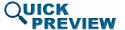• Abstract
• Authors
• Figures
• Multimedia
• References
• Cited By
• Keywords

# Circuit optimization algorithms for real-time spectrum sharing between radar and communications

The ability of radar and communication applications to share the radio spectrum will require the use of innovative agile circuit techniques for radar and communications. Reconfigurable circuits can provide real-time adjustment of operating frequency and spectral output, while maintaining system performance and maximizing power efficiency. This paper discusses recent developments in circuit optimization techniques for power efficiency and spectral performance. Optimization of a single parameter (load reflection coefficient) for multiple criteria is first addressed, followed by multiple-parameter, multiple-criteria optimizations. The use of the recently innovated Smith Tube to optimize additional parameters, such as input power and bias voltage, simultaneously with the load impedance is discussed. Optimization examples and a forward look to fast, emerging multidimensional circuit optimization techniques are provided.

SECTION I

## INTRODUCTION

Due to increased crowding of the radio spectrum, regulators have begun practicing dynamic spectrum allocation in many bands. To allow the dynamic allocation of spectrum to be performed over a larger portion of the radio spectrum, transmitter and receiver amplifier circuitry must be adaptable in real-time to meet changing operating frequency and output spectrum requirements, while being power efficient and maintaining acceptable bit-error rate (communications) and ambiguity function (radar) properties. In most cases, a spectral mask is assigned to a radar or communication transmitter output by regulators. In future protocols supporting dynamic frequency allocation, this mask may be adjustable based upon geographic and spectral proximities of nearby wireless applications.

Adaptive impedance matching is needed to facilitate changes in operating frequency and spectral output. Lu and Vaka-Heikkila have presented a micro-electrical mechanical systems (MEMS) switching scheme to construct amplifiers with adaptive output matching networks , . For many applications, frequency-agile circuits must be tunable over a broad range. An adaptive impedance tuner is presented by Deve, who demonstrates tuning from 1 to 3 GHz . Sun discusses the criticality of minimizing the number of iterations in impedance tuning algorithms . Real-time impedance matching is demonstrated in the literature , , and Qiao demonstrates a tunable amplifier using a genetic algorithm for low-power communication applications . Baylis discusses the criticality of reconfigurable transmitter power amplifiers for “solving the spectrum crisis” .

For visualization of multi-parameter circuit optimizations, the Smith Tube has been introduced as an extension of the Smith Chart     . The vertical axis can represent input power , bias voltage , or bandwidth .

This paper overviews our recent contributions in fast circuit optimizations for multiple and conflicting system objectives that will be useful in the real-time, spectrum-aware optimization of communication and radar transmitters.

SECTION II

## VECTOR-BASED OPTIMIZATION IN THE SMITH CHART FOR MULTIPLE OBJECTIVES

A vector-based algorithm for constrained optimization has been created in the Smith Chart. The load reflection coefficient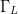$\Gamma_{L}$ can be tuned in a real-time search to maximize the power-added efficiency (PAE) while maintaining the adjacent-channel power ratio (ACPR) below a pre-specified limit . The search vector is based on estimation of the PAE and ACPR gradients in the Smith Chart from surrounding-point measurements separated by neighboring-point distance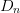$D_{n}$, as shown in Fig. 1.

Figure 2 shows the construction of a search vector based on the gradient vectors to locate the next candidate$\Gamma_{L}$ in the Smith Chart. Unit vectors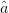$\hat{a}$ and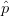$\hat{p}$ represent the optimum ACPR and PAE directions, respectively, and are calculated from the gradients.$\hat{b}$ is the bisector between these vectors. If the ACPR value at Candidate 1 is out of compliance, the search vector is constructed as follows: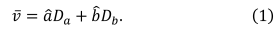TeX Source

Fig. 1. Measurement estimation of the PAE and ACPR gradients in the smith chart for the vector-based search algorithm, reprinted from . PAE and ACPR are measured at neighboring points separated from the candidate value of$\Gamma_{L}$ by$D_{n}$ in the positive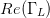$Re(\Gamma_{L})$ and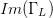$Im(\Gamma_{L})$ directions.

If the ACPR value at Candidate 1 is within compliance, the search vector is given as follows: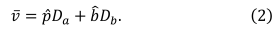TeX Source

The strengths of the vector components are given  by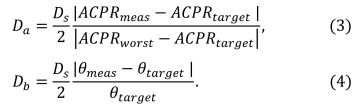TeX Source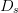$D_{s}$ is the search-distance parameter selected by the user. The measured, goal, and worst-case values of ACPR and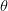$\theta$, the angle between the PAE and ACPR-PAE bisector vectors, are used to calculate the search vector components. Measurement testing of the algorithm was performed using a Maury Microwave Automated Tuner System (ATS) load-pull setup with signal generator, power meter/sensor, and spectrum analyzer from Keysight Technologies with chirp waveform excitation. The device under test was a Skyworks packaged amplifier, with the optimization goal to maximize PAE while maintaining ACPR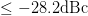$\leq-28.2\mathrm{dBc}$. Figure 3 shows that the fast search algorithm measurement results compare very well with the results extracted from traditionally measured load-pull data. These results and algorithm test results from other starting points show that between 11 and 25 measured points were necessary to locate the constrained optimum in five searches started from varying locations on the Smith Chart .

Fig. 2. Search vector construction to locate the next candidate$\Gamma_{L}$ in the search for the cases where (a) ACPR at candidate 1 is greater than the pre-determined limit value (out of compliance) and (b) ACPR at candidate 2 is less than the pre-determined limit value (in compliance). Reprinted from .
Fig. 3. (a) Fast algorithm measurement search results for the smith chart search algorithm to find the highest PAE possible while maintaining ACPR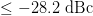$\leq-28.2\ \mathrm{dBc}$. The optimum is found with 22 measurements. The algorithm results in a constrained optimum PAE of 6.529%, ACPR = −28.2 dbc, located at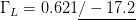$\Gamma_{L}=0.621\underline{/-17.2}$.(b) location of the constrained optimum from traditionally measured load-pull data: optimum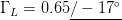$\Gamma_{L}=0.65\underline{/-17^{\circ}}$ with PAE = 6.65% and ACPR = −28.2 dbc. Reprinted from .

An expanded algorithm has been constructed that has shown success in optimizing PAE under constraints on both ACPR and delivered power . This dual-constraint situation is applicable to most communication and radar applications, because the transmitted power is very critical to receipt of the message with necessary signal-to-noise ratio (communications) or successful illumination of a target (radar).

SECTION III

## MULTI-PARAMETER, MULTI-OBJECTIVE REAL-TIME CIRCUIT OPTIMIZATION ALGORITHMS

The Smith Chart provides an optimization space to search for the constrained optimum$\Gamma_{L}$. If it is desired to optimize additional parameters simultaneously in the real-time amplifier, additional dimensions are needed in the search space. The Smith Tube (Fig. 4) allows visualization for realtime optimization of multiple circuit parameters for multiple criteria. The vector-based search uses search vectors constructed as in Fig. 2, but in the three-dimensional search space, based on the three-dimensional gradient estimations. Figure 5 shows algorithm measurement results in the Power Smith Tube. In this measurement test, it was desired to optimize$\Gamma_{L}$ and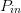$P_{in}$ simultaneously to achieve the highest PAE possible while maintaining ACPR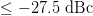$\leq-27.5\ \mathrm{dBc}$ . The constrained optimum was achieved in just 39 measurements for the three-dimensional search. A comparison of optimization from multiple starting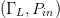$(\Gamma_{L},P_{in})$ starting combinations is detailed in . Measurement results varied from 24 to 39 experimental queries over five different tested starting points. Figure 5 shows that the endpoint from the intelligent search is very close to the constrained optimum value assessed from multiple-power load-pull data.

Fig. 4. The power smith tube, reprinted from . The vertical axis represents input power$P_{in}$, while the horizontal plane is a smith chart representing the complex load reflection coefficient$\Gamma_{L}$.

The Bias Smith Tube offers promise for simultaneous optimization of a device bias voltage with$\Gamma_{L}$ . Efforts are underway to demonstrate a fast search algorithm for the optimum in the Bias Smith Tube.

Fig. 5. (a) Measurement search performed in the power smith tube for a skyworks packaged amplifier with start and end points shown. 39 measured points were required to complete the search, resulting in PAE = 7.23% at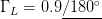$\Gamma_{L}=0.9\underline{/180^{\circ}}$ and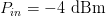$P_{in}=-4\ \mathrm{dBm}$. (b) Surface representing ACPR = −27.5 dbc (the constraint value) constructed from traditional load-pull measurements at multiple values of$P_{in}$. The optimum from the traditional measurements is PAE = 7.39% at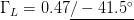$\Gamma_{L}=0.47\underline{/-41.5^{\circ}}$ and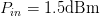$P_{in}=1.5\mathrm{dBm}$. Reprinted from 

Multidimensional Smith Tubes and multi-parameter fast searches are being explored for real-time amplifier optimization . As searches expand to greater dimensions, it will be necessary to minimize the curse of dimensionality on the search convergence speed. We have recently initialized a study comparing pattern, gradient, and simplex searches in two, three, four, and five dimensional searches using the Smith Tube framework .

SECTION IV

## CONCLUSIONS

Real-time circuit optimization searches for frequency-agile circuits will be useful in designing spectrum-aware, reconfigurable communication and radar transmitters. Fast circuit optimization algorithms will allow transmitters to change operating frequency, spectral output, and communication or detection capability while preserving power efficiency. The Smith Tube is a useful visualization tool for these optimizations, and ongoing work is exploring the use of fast searches in multidimensional Smith Tubes to simultaneously optimize multiple circuit parameters.

### Acknowledgments

This work has been funded by the National Science Foundation (Grant No. ECCS-1343316). The authors wish to thank Keysight Technologies for cost-free loan of the Advanced Design System software, and Modelithics for donation of their model libraries.

## Footnotes

No Data Available

None

## Corrections

None

This paper appears in:
Conference Date(s):
2-6 May 2016
Conference Location: